# Consider the flow net shown below: The sides of the region are groundwater divides; the top...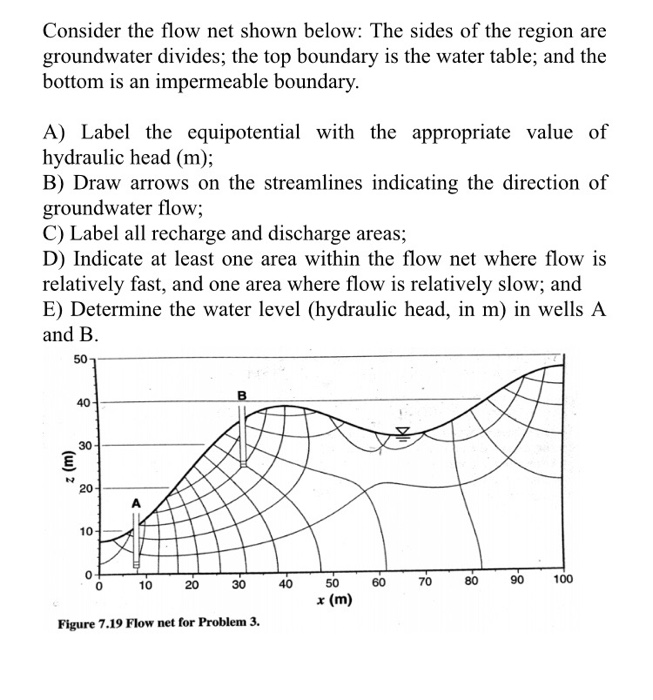Consider the flow net shown below: The sides of the region are groundwater divides; the top boundary is the water table; and the bottom is an impermeable boundary. A) Label the equipotential with the appropriate value of hydraulic head (m); B) Draw arrows on the streamlines indicating the direction of groundwater flow; C) Label all recharge and discharge areas; D) Indicate at least one area within the flow net where flow is relatively fast, and one area where flow is relatively slow; and E) Determine the water level (hydraulic head, in m) in wells A and B. 50 B 40 30 . 20 10 10 20 40 60 30 70 80 90 100 50 * (m) Figure 7.19 Flow net for Problem 3.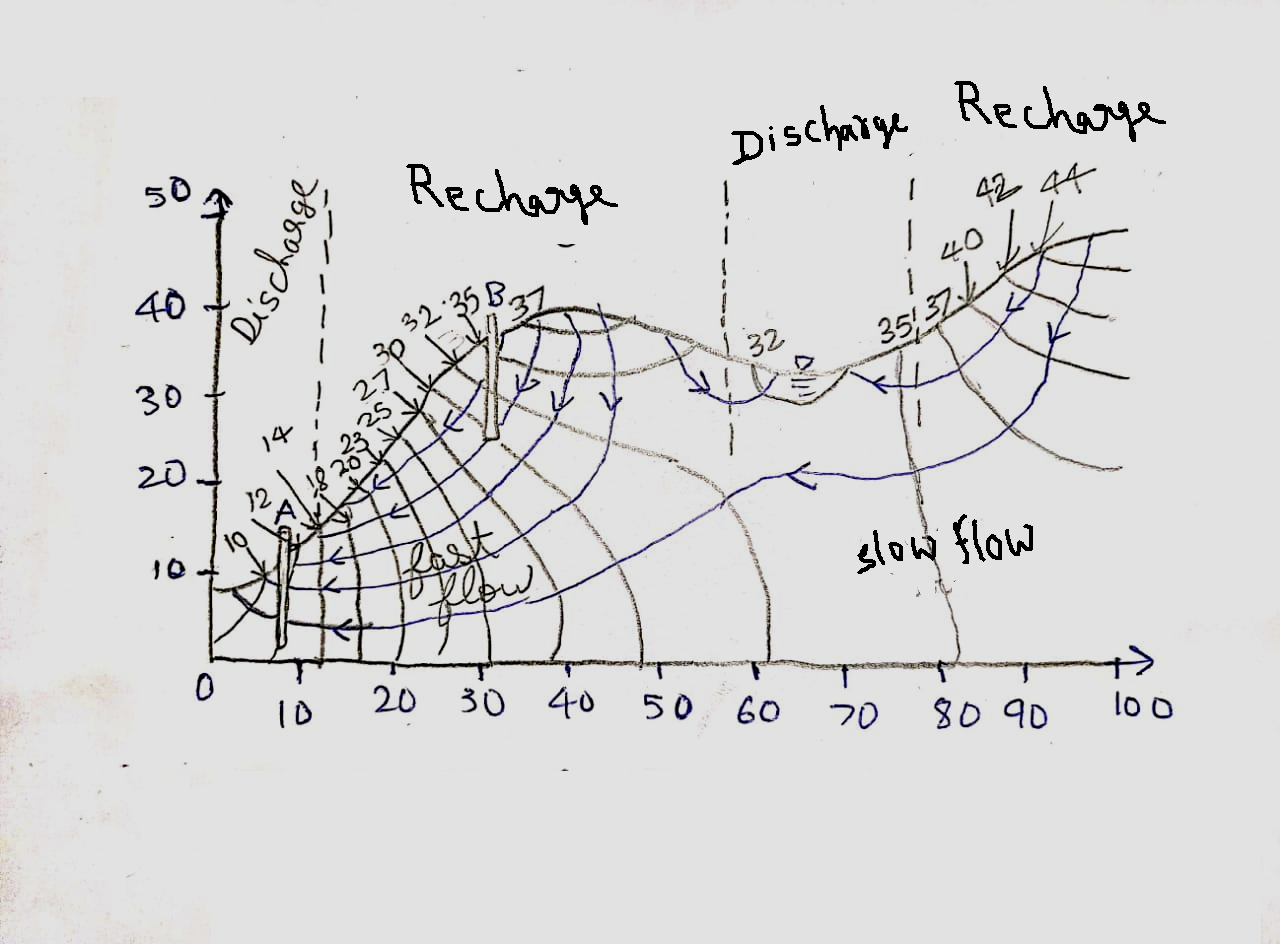The water level at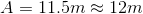The water level at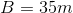Answers are directly obtained from the graph.

Hydraulic head of equipotential lines are the value corresponding to y axis.

The direction of flow is from higher potential to lower potential.

The recharge and discharge is according to the direction of flow of equipotential lines, i.e. start points of equipotential line gives recharge and end points give discharge.

Faster will be the flow at densely spaced portion of graph and the other slower.

The water level of A and B are the value of y axis corresponding to the lop boundary of water table.

#### Earn Coin

Coins can be redeemed for fabulous gifts.

Similar Homework Help Questions
• ### Problem 4 (20 points) Consider the flow net shown below: The sides of the region are...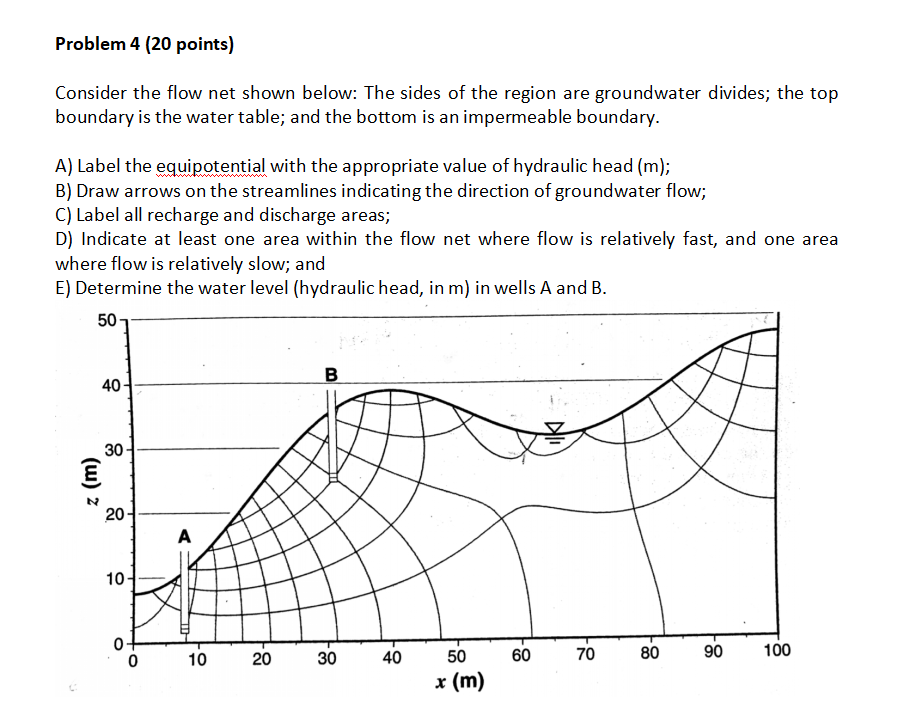Problem 4 (20 points) Consider the flow net shown below: The sides of the region are groundwater divides; the top boundary is the water table; and the bottom is an impermeable boundary. A) Label the equipotential with the appropriate value of hydraulic head (m); B) Draw arrows on the streamlines indicating the direction of groundwater flow; C) Label all recharge and discharge areas; D) Indicate at least one area within the flow net where flow is relatively fast, and one...

• ### For Following Multiple Choice All Correct Answers Which Applied To Each Question. 9. A flow net:...

For Following Multiple Choice All Correct Answers Which Applied To Each Question. 9. A flow net: is a diagram of equipotentials and streamlines. depicts steady groundwater flow in two dimensions. may be used to quantify groundwater flow. 10. When the hydraulic conductivity varies from point to point within an aquifer, the aquifer is said to be: homogeneous heterogeneous isotropic anisotropic 11. The Laplace equation: is a mathematical statement of the law of conservation of mass combined with Darcy’s law. may...

• ### 2. Parallel drainage canals are installed in a rectangular unconfined aquifer. They fully penetrate the aquifer and are 50 m apart. The elevation difference between the land surface and the bottom of...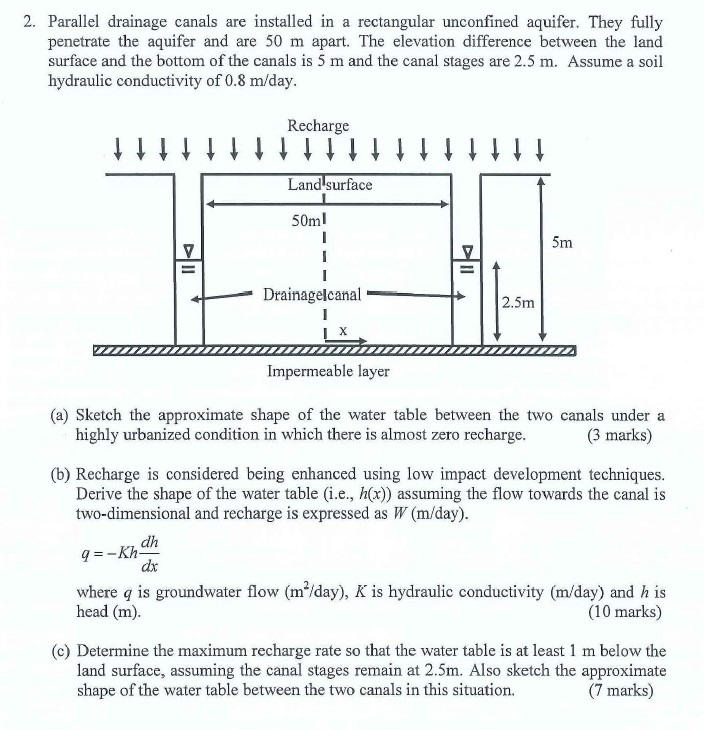2. Parallel drainage canals are installed in a rectangular unconfined aquifer. They fully penetrate the aquifer and are 50 m apart. The elevation difference between the land surface and the bottom of the canals is 5 m and the canal stages are 2.5 m. Assume a soil hydraulic conductivity of 0.8 m/day Recharge Land surface 50ml Drainagelcanal 2.5m Impermeable layer (a) Sketch the approximate shape of the water table between the two canals under a highly urbanized condition in which...

• ### Value for transmissivity is 185，location is B，flow rate is 20 Question 1: No-flow boundary conditions are implemented by: Question 2: Flow Calculation with no abstraction or rech...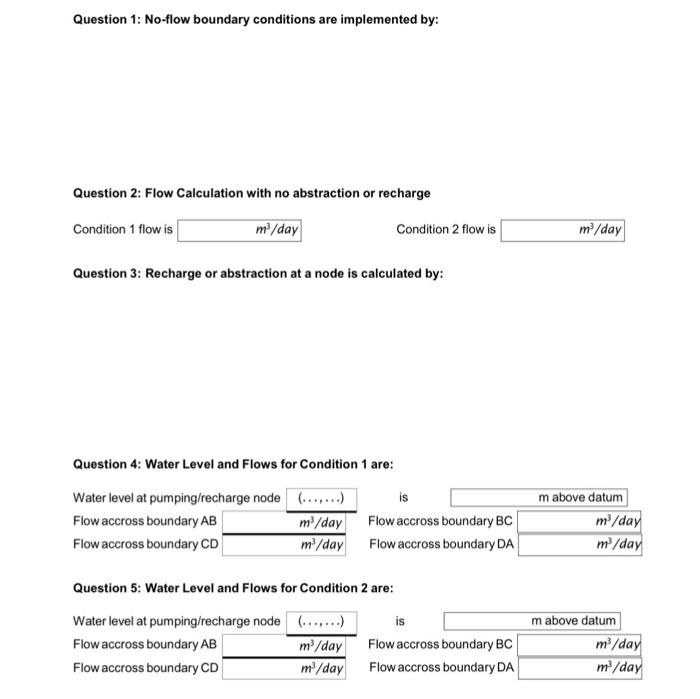Value for transmissivity is 185，location is B，flow rate is 20 Question 1: No-flow boundary conditions are implemented by: Question 2: Flow Calculation with no abstraction or recharge m2/day m/day Condition 1 flow is Condition 2 flow is Question 3: Recharge or abstraction at a node is calculated by: Question 4: Water Level and Flows for Condition 1 are: Water level at pumping/recharge node Flow accross boundary AB Flow accross boundary CD ..,..) is m3/dayFlow accross boundary BC m/dayFlow accross boundary...

• ### Q2) A canal of trapezoidal section has bed width of 8 m and bed slope of...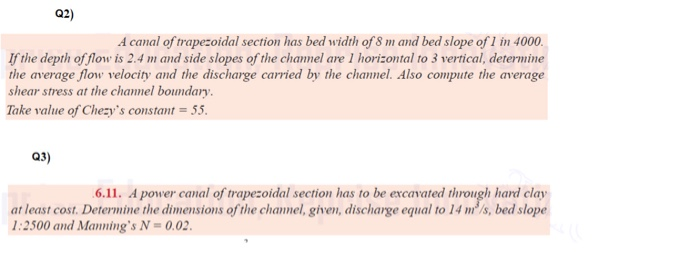Q2) A canal of trapezoidal section has bed width of 8 m and bed slope of 1 in 4000. If the depth of flow is 2.4 m and side slopes of the chamel are 1 horizontal to 3 vertical, determine the average flow velocity and the discharge carried by the channel. Also compute the average shear stress at the channel boundary. Take value of Chezy's constant = 55. Q3) 6.11. A power canal of trapezoidal section has to be excavated...

• ### 3. The flow net shown below illustrates flow conditions beneath a concrete dam featuring an upstream...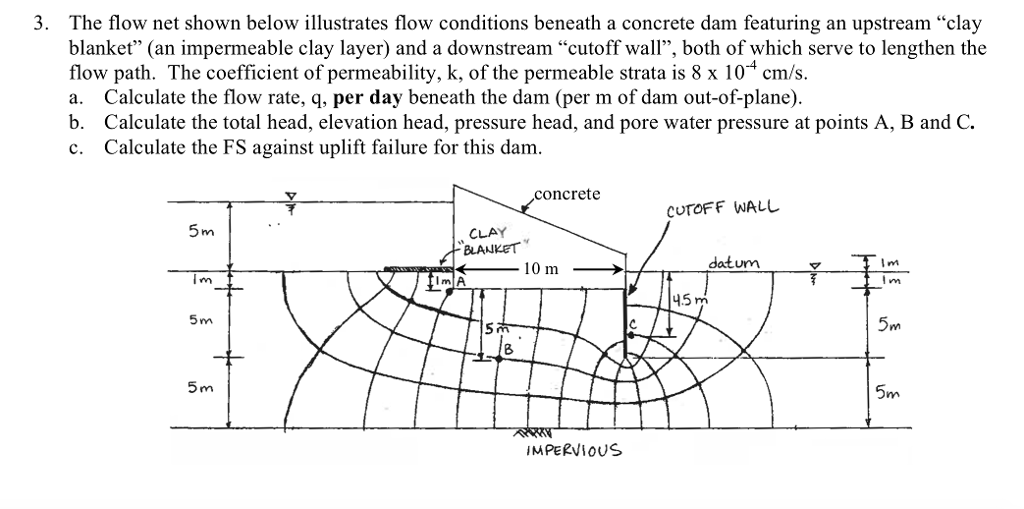3. The flow net shown below illustrates flow conditions beneath a concrete dam featuring an upstream "clay blanket" (an impermeable clay layer) and a downstream "cutoff wall", both of which serve to lengthen the flow path. The coefficient of permeability, k, of the permeable strata is 8 x 10 cm/s. a. Calculate the flow rate, q, per day beneath the dam (per m of dam out-of-plane). b. Calculate the total head, elevation head, pressure head, and pore water pressure at...

• ### *need the uplift force Problem 5 Consider the flow net shown below. The sandy soil has...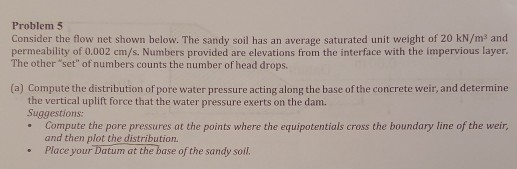*need the uplift force Problem 5 Consider the flow net shown below. The sandy soil has an average saturated unit weight of 20 kN/m and permeability of 0.002 cm/s. Numbers provided are elevations from the interface with the impervious layer. The other "set" of numbers counts the number of head drops. (a) Compute the distribution of pore water pressure acting along the base of the concrete weir, and determine the vertical uplift force that the water pressure exerts on the...

• ### 25 marks (allow 30 minutes) QUESTION3 The Figure below (Figure 3) shows a pipe system with a valve and two reservoirs. A pump transports a constant flow rate of Q # 0.1 mls of water from reser...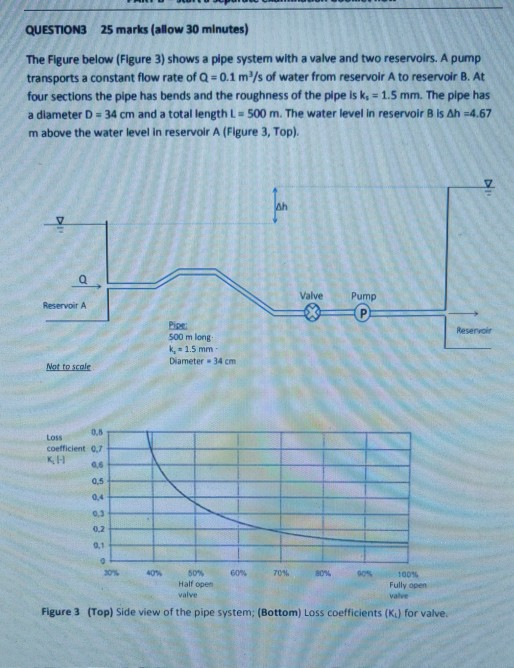25 marks (allow 30 minutes) QUESTION3 The Figure below (Figure 3) shows a pipe system with a valve and two reservoirs. A pump transports a constant flow rate of Q # 0.1 mls of water from reservoir A to reservoir B. At four sections the pipe has bends and the roughness of the pipe is k, 1.5 mm. The pipe has a diameter D 34 cm and a total length L 500 m. The water level in reservoir B is...

• ### QUESTION3 25 marks (allow 30 minutes) The Figure below (Figure 3) shows a pipe system with a valve and two reservoirs. A pump transports a constant flow rate of Q 0.1 m3/s of water from reservoi...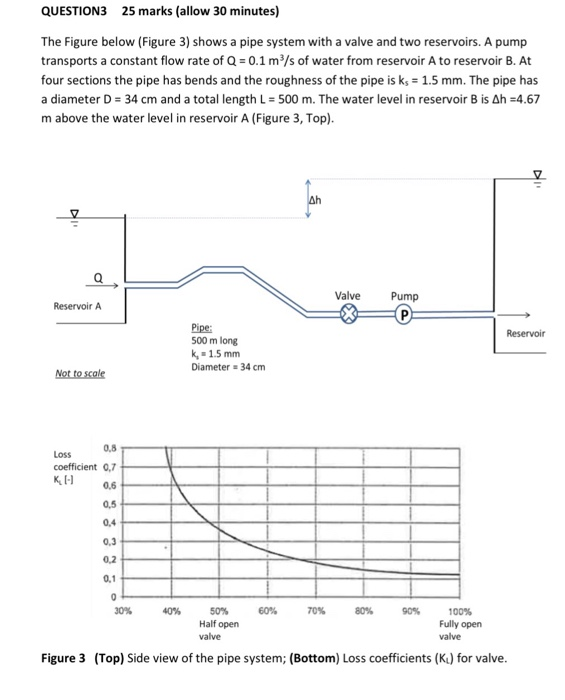QUESTION3 25 marks (allow 30 minutes) The Figure below (Figure 3) shows a pipe system with a valve and two reservoirs. A pump transports a constant flow rate of Q 0.1 m3/s of water from reservoir A to reservoir B. At four sections the pipe has bends and the roughness of the pipe is ks 1.5 mm. The pipe has a diameter D 34 cm and a total length L = 500 m. The water level in reservoir B is...

• ### please help with how the flow chart will look like? thank you! Synthesis of n-Butyl Bromide...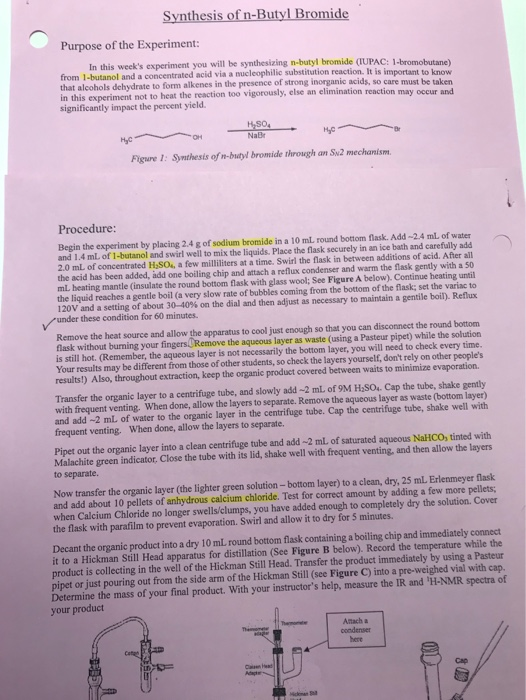please help with how the flow chart will look like? thank you! Synthesis of n-Butyl Bromide Purpose of the Experiment: In this week's experiment you will be synthesizing n-butylbromide (IUPAC 1-bromobutane from 1-butanol and a concentrated acid via a nucleophilie substitution reaction. It is important to know that alcohols dehydrate to form alkenes in the presence of strong inorganic acids, so care must be taken in this experiment not to heat the reaction too vigorously else an elimination reaction may...